## Main

The standard model is the theory that describes particles and their fundamental interactions, although without taking into account gravitation. However, this model is known to be incomplete, which has inspired searches for physics beyond the standard model, such as tests of CPT invariance that compare the fundamental properties of matter-to-antimatter equivalents at the lowest energies and with the greatest precision12,13,14,15. For leptons, for example, the magnetic anomalies of electron and positron were compared with a fractional uncertainty of about 2 parts per billion4, and by applying similar techniques to protons and antiprotons, the resulting g-factor (a proportionality constant which links the spin of a particle to its magnetic moment) comparison reached a precision of 4.4 parts per million8. We are planning to improve this measurement by at least a factor of a thousand16,17. In this context, we recently reported the most precise and first direct high-precision measurement of the proton magnetic moment, with a fractional precision of 3.3 parts per billion18. Complementary to these efforts, spectroscopic comparisons of hydrogen and antihydrogen are underway; recent progress has been made at CERN13,19. The most precise test of CPT invariance with baryons is the comparison of the proton and antiproton charge-to-mass ratios. By measuring the cyclotron frequencies vc = (qB0)/(2πm) of single trapped antiprotons and H ions in a Penning trap with magnetic field B0, the TRAP collaboration7 achieved a fractional precision of 90 parts per trillion.

In our measurements we also compare the cyclotron frequencies of a single antiproton and an H ion. H is used as a proxy for the proton; the negative charge facilitates the experiment by eliminating the need to invert trap voltages. Our advanced Penning trap system enables adiabatic particle exchange within 15 s, which is much faster than in previous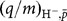comparisons. Our fast exchange rate allows for individual particle-to-antiparticle comparison cycles of only four minutes. This high-precision mass spectrometry method enabled us to perform about 6,500 direct frequency ratio comparisons within a total measuring time of 35 days. Moreover, our measurements have been carried out in thermal equilibrium with the detection system at 5.2(1.1) K, where systematic frequency shifts are small20.

Our cryogenic Penning-trap system, which consists of a measurement trap and a reservoir trap, is shown in Fig. 1. It is mounted in the horizontal bore of a superconducting magnet at B0 = 1.946 T, the axis of the magnet being oriented at 60° with respect to the Earth’s rotation axis. Both traps have an inner diameter of 9 mm and are arranged in the five-electrode orthogonal and compensated design discussed in ref. 21. Transport electrodes connect the individual traps; they allow for fast adiabatic particle shuttling along the trap axis. The entire assembly is mounted in an indium-sealed cylindrical vacuum chamber with a volume of 1.2 litres. Once cooled to 4 K, ultralow pressures are reached, and thus antiproton storage lifetimes of more than a year are achieved. To measure the particle’s oscillation frequencies by image current detection22 we used highly sensitive superconducting resonant detection coils23. The measurement trap detector is operated at a resonance frequency of vres = 645,262 Hz, has an inductance of 1.72 mH, and a quality factor of 11,300.Figure 1: Schematic of the measurement and the reservoir Penning traps.

Antiprotons are delivered in bunches by the Antiproton Decelerator of CERN; the antiproton bunches also release hydrogen from our degrader structure. H ions are produced either by asymmetric dissociation of H2 or by electron capture (a detailed study of the production mechanism has yet to be performed). Typically 100 to 350 cold antiprotons and about a third that number of H ions are prepared per ejection from the Antiproton Decelerator. From this particle cloud we extract a single antiproton and keep it in the centre of the trap, as well as an H ion, which is parked in the downstream park electrode. The other particles are shuttled to the reservoir trap. In case particles are lost during experiments, the measurement trap is reloaded from this reservoir.

In charge-to-mass ratio comparisons cyclotron frequencies vc are measured. We use the invariance theorem24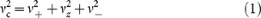which relates the characteristic trap frequencies v+, v and vz to vc. Comparisons of the antiproton-to-H charge-to-mass ratio are equivalent to a direct antiproton-to-proton comparison, but systematic shifts caused by polarity switching of the trapping voltages are avoided. The mass of the negative hydrogen ion is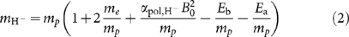where me /mp is the electron-to-proton mass ratio25,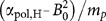is the polarizability shift9, Eb/mp is the electron binding energy26 and Ea/mp is the electron affinity of hydrogen27. If CPT invariance holds, the expected cyclotron frequency ratio is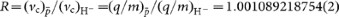, the precision being limited by the accuracy of our knowledge of the proton mass28.

A detailed measurement cycle is shown in Fig. 2a. Our measurements are triggered by the antiproton injection into the Antiproton Decelerator; this avoids systematic ratio shifts induced by beats between the measurements and ambient field fluctuations caused by the Antiproton Decelerator cycle. Immediately after the injection trigger, the magnetron motion of the antiproton is cooled for 10 s (ref. 18). Then the antiproton’s axial frequency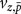is measured, followed by a determination of the modified cyclotron frequency at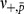= 29.656 MHz, which is extracted from a sideband measurement as described in ref. 18. These two measurements take 30 s and 48 s, respectively. The particle’s magnetron frequency is determined by evaluating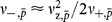= 7.02 kHz, and thus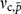≈ 29.663 MHz is obtained. Next, we ramp the trapping potential from the configuration shown in Fig. 2b to that shown in Fig. 2c, and adjust the trapping voltage by 5 mV to tune the axial oscillation frequency of the H ion into resonance with the superconducting detector. Afterwards, we perform similar measurements with the hydrogen ion to determine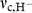≈ 29.635 MHz. Thus, a single ratio comparison takes exactly two Antiproton Decelerator cycles, corresponding to a typical measuring time of 220–240 s. To obtain from all performed measurements the final experimentally determined frequency ratio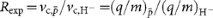, we reject those data points measured when magnetic field changes caused by activities in the Antiproton Decelerator accelerator hall were observed. These changes are identified by an array of giant magneto resistance, Hall and flux-gate magnetic field sensors.

Subsequently the ratios are processed as follows. To remove systematic ratio shifts caused by the intrinsic magnetic-field drift 1/B0 × (ΔBt) = −5(1) × 10−9 per hour of our superconducting magnet, we compute the antiproton cyclotron frequencies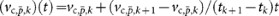, where k is the index of individual measurements. Subsequently we evaluate the upper ratio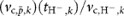, where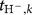is the centre time of the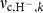determination. In addition, the reciprocal ratios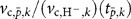are evaluated in a similar way. From the processed data we extract the mean of the frequency ratio by performing a maximum-likelihood fit of a Gaussian distribution to the upper ratio as well as to the reciprocal results and calculating the average of both.

To estimate the uncertainty of the mean we evaluate the correlation matrix of the extracted ratios and calculate the standard error of the cross-correlated data. This avoids underestimation of the error caused by frequencies which are, owing to the linear averaging approach, used in multiple ratios. All measured upper ratios are shown in Fig. 3a and b. The full time sequence is shown in Fig. 3a, and the results projected to a histogram are shown in Fig. 3b. Breaks in the time sequence are due to maintenance of the apparatus or systematic measurements. In Fig. 3c and d, the power spectrum density and the Allan deviation of all measured data points are shown. The mean of the power spectrum density is constant, while a linear fit to the double-log plot of the Allan deviation gives a slope of α = −0.501(2). This confirms the Gaussian white-noise nature of the ratio fluctuations and justifies our data analysis.

For further verification we evaluate the Allan deviation of our cyclotron frequency measurements, which is shown in Fig. 3e as the red data points. A fit of a linear drift of −5(1) parts per billion per hour (dashed line in Fig. 3e), a white-noise contribution with a root-mean-square width of 160(15) mHz per cycle (dash-dotted line in Fig. 3e), and a random walk generating a Gaussian distribution of 220(20) mHz per cycle (dotted line in Fig. 3e) reproduce the experimental results. From this model we simulate data, add random offsets Δr and blind-analyse the simulation data. Within error bars the offsets Δr are reproduced, which independently justifies our data analysis. The antiproton-to-H mass ratio extracted from this data evaluation is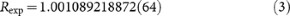To further check both the data analysis and the experiment we also evaluate the cyclotron frequency ratios for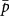-to-and H-to-H instead of-to-H, but measured within subsequent cycles. For these direct comparisons of identical particles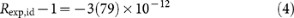is obtained, which is consistent with 1. The increased statistical error in the second case is caused by the random walk in the magnetic field, which leads to slightly higher ratio fluctuations owing to the doubled time interval between subsequent measurements of identical particles.

Several systematic corrections enter into the measured antiproton-to-H charge-to-mass ratio Rexp. The dominant systematic shift is related to particle exchange and the 5-mV detuning of the trapping voltage, which is required to tune the axial oscillation frequencies of both particles to the centre of the axial detector. Slightly different contact and offset potentials as well as machining imperfections are present at each individual trap electrode. Thus, the change of the ring voltage causes a relative shift of the antiproton-to-H equilibrium position. In the presence of a magnetic gradient term of B1 = 7.58(42) mT m−1 (where B1 is the strength of the magnetic gradient) the cyclotron frequenciesand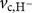are hence measured at slightly different magnetic fields, leading to a systematic ratio shift. In our measurement, the adjustment of the trapping voltage shifts the H ion towards lower magnetic fields, and Rexp has to be corrected by −114(26) parts per trillion. A detailed discussion of this dominant systematic shift is provided in the Supplementary Information.

The systematic uncertainty arises from the uncertainty in the determination of the offset voltages and the magnetic gradient B1. In addition to the particle position, the magnitude of the octupolar correction C4 of the trapping potential20 also changes when the trap voltage is adjusted. The resulting ratio correction is at −3(1) parts per trillion, the error being due to uncertainties extracted from potential theory and the determination of the axial mode temperature Tz of the particle. Eventually, the stability of our rubidium frequency reference contributes a systematic scatter of 3 parts per trillion per ratio comparison. In summary, the ratio has to be corrected by −117(26) parts per trillion, leading to our final result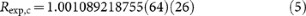which corresponds to an antiproton-to-proton mass ratio of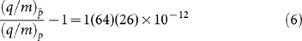and is in agreement with CPT conservation.

In the framework of the standard model extension developed by ref. 29 a figure of merit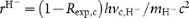is derived, which characterizes the sensitivity of our measurement with respect to the CPT-violating terms added to the standard-model Lagrange density. Our result sets a new limit of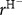< 9 × 10−27. This exceeds the previous limit by a factor of four, and probes the standard model at an energy scale of 8 atto-electronvolts. In terms of energy sensitivity our result is the most stringent test of CPT invariance with baryonic antimatter performed so far.

In addition to the above discussion, our high data accumulation rate enables the search for sidereal variations, which might be mediated by cosmological background fields. To this end, the data set is processed using lock-in filter and Allan-deviation-based data analysis. A diurnal variation of the results would appear as a peak in the lock-in spectrum at a period of the sidereal day, which is 86,164.1 s. We do not observe such an indication and from our data analysis we conclude that the amplitude of any diurnal variation in Rexp is <0.72 parts per billion at the 0.95 confidence level.

By following the arguments of ref. 11 our measurements can be interpreted as a test of weak equivalence. If the proton-to-antiproton charge-to-mass ratios are identical in the absence of a gravitational field, then the particle and antiparticle cyclotron clocks have the same frequencies. However, if matter respects weak equivalence while antimatter experiences an anomalous coupling to the gravitational field, the cyclotron frequencies of the two particles will experience different gravitational redshifts when moved to the surface of the Earth. We follow ref. 11, in which a possible gravitational anomaly acting on antimatter is expressed by a parameter αg, which modifies the effective newtonian gravitational potential U to give αgU. This contributes a possible difference in the measured cyclotron frequencies of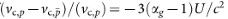Thus, our measurement sets a new upper limit of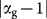< 8.7 × 10−7.